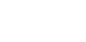## How to Use Math to Solve Problems

### IntroductionMath literacy for the information age means much more than the ability to do the simple calculations of arithmetic. In Workplace Basics: The Essential Skills Employers Want, Anthony Carnevale includes the following categories for computation in a technological workplace:

Quantification   -- the ability to read and write numbers, count, put numbers in sequence, recognize when one number is larger than another

Computation   -- the ability to add, subtract, multiply and divide with single- and multiple-digit whole numbers, mixed numbers, fractions and decimals

Measurement and Estimation   -- the ability to take measurements of time, temperature, distance, length, volume, height, weight, velocity, speed, to use and report measures correctly; when exact measurement is impractical or unnecessary, the ability to apply simple estimating techniques

Problem Solving   -- the ability to use the above skills in real-life situations; recognizing problem, gathering information, deciding appropriate mathematical steps, computing answers

Comprehension   -- the ability to draw on knowledge and skill in the areas of equivalents, organizing data, algebra and geometry

Math skills are necessary not just for performing on the job, but also for day-to-day survival. You have to understand, even though you may not have to use, all of these mathematical principles to be able to make good decisions about your work and your social, leisure and personal goals.

In this article, we will describe and define several areas of day-to-day activities that will require you to have a minimum level of mathematical understanding and skill. We will give you a broad sampling of links to connect with the ample math resources available on the Web.

This material is based on coursework developed by the Consortium for Mathematics and Its Application in the 26 half-hour TV programs and accompanying book called For All Practical Purposes: Introduction to Contemporary Mathematics.

Learning Goals

At the completion of all of the segments of this article, you should be able to:

• name the chief categories of math literacy for today's workplace
• list and describe five areas in which modern society is impacted by mathematical concepts
• use the included Internet links to get additional information about the topics of mathematics and real-life problem solving